# Nothing but (neural) net

We start a new series on neural networks and deep learning. Neural networks and their use in finance are not new. But are still only a fraction of the research output. A recent Google scholar search found only 6% of the articles on stock price price forecasting discussed neural networks.1

Artificial neural networks, as they were first called, have been around since the 1940s. But development was slow until at least the 1990s when computing power rapidly increased. Through this period the architecture and algorithms to build and train networks proceeded steadily. Nonetheless, it wasn’t until 2015 or so with the release of Keras (a high-level deep Learning API) that the floodgates opened. Multiple implementations by various providers were released quickly and there are now a number of other deep learning libraries, including TensorFlow by Google and PyTorch by Facebook.

How these all work are beyond the scope of this post, though we hope to touch on all of those libraries within this series. The main point is that with open-source software it is relatively straightforward (though not necessarily easy!) for an individual to build, train, and deploy a deep neural network for all sorts of machine learning problems: natural language processing, computer vision, musical composition, etc. In fact, it would seem deep learning and artificial intelligence (AI) are everywhere.

A cursory read of the applications in use today vs what was possible even a few years is truly astounding, leading some to foresee the singularity and others to warn of a dystopian future not unlike an 1980s action classic, starring Hollywood’s favorite Austrian bodybuilder.

Futurists we are not. As this is blog is about data science and investing, we’d prefer to ask a few simple questions:

• Can the use of neural networks improve the investing process?
• If so, how?
• If not, why not?

It should be relatively apparent that the first question is deceptively complex. Unpacking it implies a bunch of corollary questions or, at least, the need to define what we mean. That is,

• How are we using neural networks? As forecasting tools? As risk mitigation tools?
• Which neural network (architecture) should we use? Simple, multi-layer perceptron, deep, convolutional, recurrent, LSTM…?
• Which library?
• And of course, the biggest question, how should we define “improve”? Better forecasts, better risk-adjusted returns, lower transaction costs, better implementation?2 And better relative to what? Buy-and-hold, other algorithms, etc.

We obviously won’t answer most of these questions now. For this post, we’ll introduce the neural network concept and start to show some of the results it can produce. Warning! The structure, implementation, and output of neural networks is complicated and complex. Truly understanding them requires a lot of effort. Explaining them thoroughly does too. We’re more concerned with understanding the results. Most people know how to drive without knowing how an internal combustion engine works. As such, we’ll likely tread a fine line between irritating folks that really understand neural networks (by forgetting something) and frustrating those that don’t (by failing to explain something else). Apologies in advance.

Let’s move on. What is a neural network? Even though the concept is patterned on neurons and synapses, neural networks look very little like a true biological neuron. We see them more or less like matrices that get manipulated, updated, and transformed.

At the most basic level, there’s a “perceptron”. It takes inputs, applies a weighting scheme to those inputs, and then uses an activation function that transforms the aggregated weights and inputs into an output that is supposed to approximate whatever it is you’re trying to forecast.

Graphically, it’s often shown by the following image.3 The lines represent the connections from the inputs into the output and the weights. The activation function is implied or not used.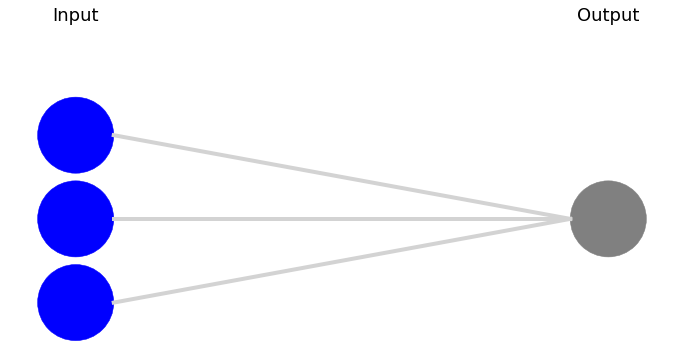In math terms it looks something like this: $$y = \phi(\Sigma w_{i}x_{i} + b)$$

where: $$\phi$$ is the activation function, $$x$$ is the input, $$w$$ are the weights, and $$b$$ is the bias term. The $$\Sigma wx$$ is the summed product of the weights and inputs. The bias term is there so that the model doesn’t bounce around randomly.

For the mathematically inclined, the part inside the $$\phi$$ function will look very much like a linear equation. We won’t go into detail about what $$\phi$$ actually is since it varies depending on the task, the structure, or architecture, of the neural network, and the type of performance one wants.4 But what it does is this: transforms the weights and inputs to match the type of output we’re trying to predict. For example, if our input was a bunch of real numbers but our labels, or desired output, were binary, we’d need some sort of function to transform the real number output into a binary one without losing too much information. However, if you need a real number, you may not even use an activation function at the output step.

Unfortunately, a simple, single-layer perceptron is too simple. It often can’t find solutions to simple problems we can solve with pencil and paper.5 But researchers found that if you stack those perceptrons on each other (i.e., multi-layer perceptrons or MLP) and allow them to interact, things really get cooking!

For an MLP, the output of one layer of perceptrosn becomes the input of another layer, often called a hidden layer. And if one layer is good, why not fifty? If a few neurons are good, why not hundreds? Graphically, it looks like this with the $$\phi$$ function in parentheses. The actual activation functions aren’t relevant for this post, but we include the to show that they aren’t necessarily the same for each layer.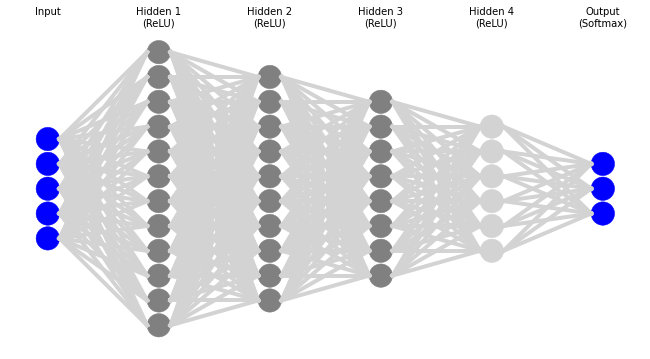Mathematically, that ends up looking something like the following:6

\begin{align} h^{} &= \phi^{}(W^{}x^{} + b^{})\\ h^{} &= \phi^{}(W^{}h^{} + b^{})\\ h^{} &= \phi^{}(W^{}h^{} + b^{})\\ h^{} &= \phi^{}(W^{}h^{} + b^{})\\ y &= \phi^{}(W^{}h^{} + b^{}) \end{align}

Where $$W^{[i]}$$ is a matrix of weights as opposed to the vector—$$w_{i}$$—from above, since each input will go into more than one neuron.

Hopefully, this isn’t complete gobbley gook. If you start from the first function—$$\phi^{}(W^{}x^{} + b^{})$$—you can see that the output of that function ($$\mathbf h^\mathbf {}$$) becomes the input of the other ($$\phi^{}(W^{}\mathbf h^\mathbf {} + b^{}$$). If you’re beginning to feel your eyes glaze over and head spin, wait! There’s more.

It’s not enough that we’ve gone through all this to reach some final output. We need to check that output against what we’re actually trying to predict. Surprise, surprise, our output is likely to be off (often astonishingly so). That means, we’ll need to go back and tweak the weights at each layer and neuron and start from the beginning all over again. This step is called backpropagation and involves both calculus and iteration.7 Once we’ve run the whole thing again, we check it against our desired output and continue re-running backpropagation, tweaking the weights, and then feeding the new weights forward until we’re satisfied or need a stiff adult beverage.

Each pass backward and forward is called an epoch and is like a microcosm of the machine learning process: train, validate, revise, repeat. As this happens automatically (thanks to the complex combination of algorithms and architecture often producing remarkably accurate results), it’s easy to see why some call this (perhaps hyperbolically) artificial intelligence.

There’s no ghost there, however. A human’s directing the machine, deciding on the architecture, how fast the neural network learns, how many times it must repeat its calculations and a bunch of other tuning knobs called hyperparameters.

If you’re still with us, you’re probably asking yourself a couple questions:

• Where do the weights and bias terms come from?
• Why does a neural network (NN) work?
• What does this have to do with investing?

The weights and biases are initially chosen at random, using some underlying distribution assumption.8 This is pretty remarkable when you think about it. You might have no idea what the appropriate weights should be, but through computational power, calculus, and the right architecture—that is, the structure of the hidden layers—the computer figures out how to tell a long-haired Persian from a Pekingese.

Why a NN works is probably better reformulated into, why is a NN able to achieve better accuracy than other algorithms? For many years, it didn’t. Many diverse elements—particularly backpropgagation—needed to be discovered, combined, and then applied efficiently. Nonetheless, NNs work broadly because trial-and-error works. If we only had one attempt at riding a bicycle to prepare for a race, the Tour de France would probably look more like slapstick than elite athleticism.

By instantiating hundreds or thousands of connections and interactions, the neural network approximates how neuroscientists believe the brain works. When we learn arithmetic or how the longbow won the Battle of Hastings, different neurons in the brain are activated and activate one another to the point that connections are created and strengthened. Activate often enough, and the patterns and resiliency of those patterns perpetuate sufficiently to allow us to remember automatically that Hastings was in 1056, er 1066.

Computers are also super good at producing massive amounts of minute trial-and-error attempts in a short fraction of time. So that helps a lot too.

What the heck does this have to do with investing? If algorithms like linear regression are used to inform investment decisions, often successfully, why not apply a more sophisticated algorithm? That’s a sufficient enough reason. But we believe—though have yet to prove—that a neural network could yield a better model of the market.

The fundamental law of finance might be that a risk asset’s price must equal the discounted value of its future cash flows—if it generates cash—and the market clearing intersection of supply and demand. But there has to be actors who have (differing) views of value, supply, and demand for all this to obtain. A neural network might be able approximate a simplified version of market participants expressing views (the weights) and biases (the bias, hehe) about an asset. But we’re way ahead of ourselves.

Let’s step back and look at a simple example, the Hello World! of trend-following and tactical allocation— the long-term simple 10-month moving average on the S&P 500.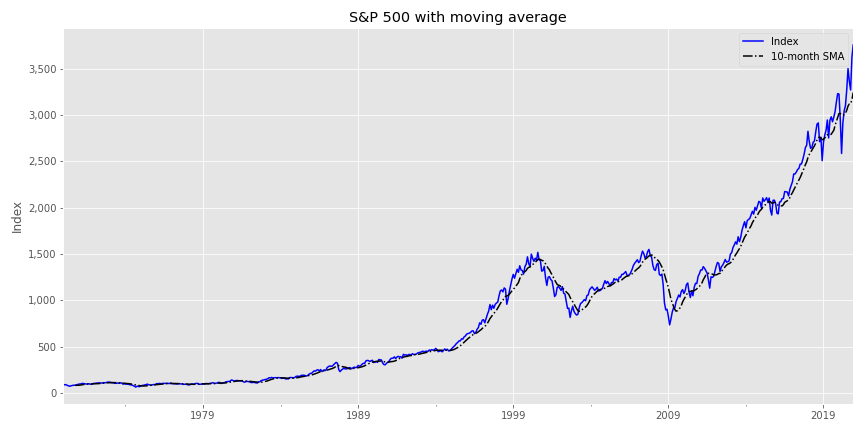Investors use the moving average to give a rough gauge of trend or signal. If the stock is above the moving average we’re in an uptrend and vice versa. When the stock crosses above the trend, BUY!, when it crosses below, SELL! Even fundamental investors will look at a chart that often includes some moving average. If this simple metric is not a basis for one’s investing decisions, it still plays a role. The neurons are firing as you look at the chart and process the information.

Let’s build a relatively simple neural network with one hidden layer populated with ten neurons. We’ll run the forward and back passes 20 times or epochs. The architecture will look something like the following.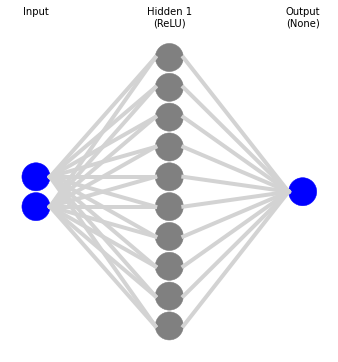The two blue inputs (features) are the current month end price and the 10-month simple moving average price. The gray neurons are the hidden layer, the single blue output (label) is the ending price in the following month. We’ll normalize all these prices according to the last ten months average and standard deviation. We’ll split the data into a training set (1970 to 1991), validation set (1991 to 2000), and a test set (2001 to 2020). Clearly, this is a simple example, wouldn’t even count as a worthy research application, and is unlikely to produce much of a good forecast. It’s a toy example to build the intuition. As we train the model, we store the results of the loss function at the end of each epoch. We can then graph of the loss function—root mean-squared error (RMSE)—to see how the model progresses.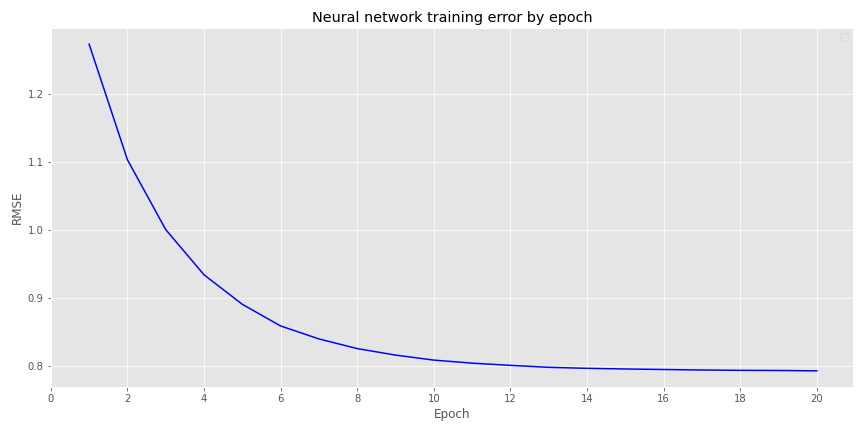As one can see the curve declines nicely over the twenty epochs, as it should do. Recall, after each epoch, the algorithm moves back through the network to tweak the weights. Notice, however the RMSE is quite large (ranging from above 1.3 to below 0.8), especially in relation to the data, which has been normalized to have a mean of zero and a standard deviation of one for each successive 10-month period.

Since nothing seems to have gone awry, We now want to see how well the model generalizes on new data, the validation set. This is essentially a test set we get to see, allowing us to tune the model better without snooping the actual test data, which we should only look at once we’ve got a model we think might work.

We run the algorithm again, but record the loss function on the validation data as well. What are we looking for in the validation data? First off, we want to examine the loss function produced by applying the model’s weights to the validation data. The graph can then tell us of how well the model generalizes. Does the validation loss trend down with the training loss (good) or does it flatten out much earlier (bad). Does the validation loss hug the training loss (good) or give it the cold shoulder (bad)?

Note: even though we get to “see” the validation data, it doesn’t affect the calculations that produce the weights and biases associated with the model, so it’s not snooping per se. Of course, anyone can pull up a long-term chart, so we’re all snooping to some degree. The only true test set is tomorrow!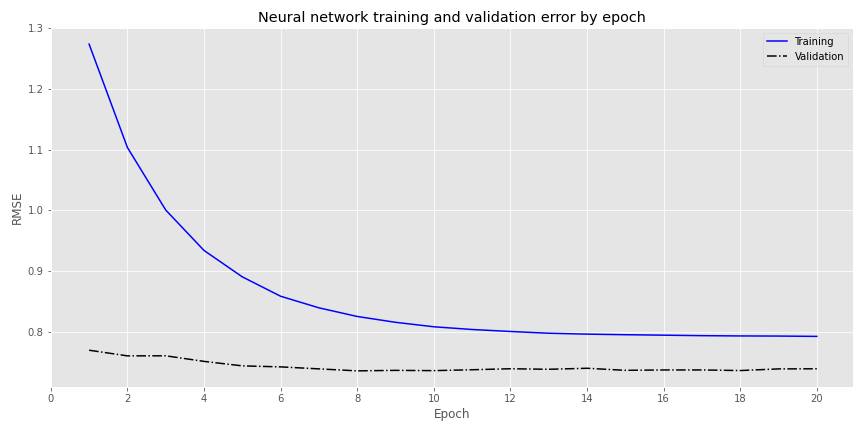Wow! Look at how much lower the error is for the validation set. Those neural networks sure are something else!

Sadly, this result is due to luck. The validation set should have a higher error rate than the training set because even though we want a model to generalize well on data it hasn’t been trained on, it would be odd for it to produce a lower error. That would be like expecting bad inputs to produce better outputs.9

This presents us with a problem. If the validation period is such that it will make the training model look incredible, we need to figure out a way to alter the data to remove the regime effects or test a different hypothesis. Solving that puzzle will be left for another post. But we can address it roundaboutly [sic!], by asking whether a different algorithm—linear regression, for example—produces similar results. This will also afford us the opportunity to show how a NN can approximate the output of a linear regression.

As we noted above, the weights and biases formula looks very similar to a linear model. One can get very close to the output of linear model using a simple NN (a single layer perceptron in fact!). In the chart below, we plot the loss of a simple NN along with a horizontal line for the RMSE produced by a linear regression.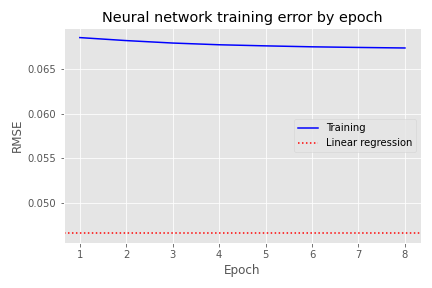We see that after six epochs the simple NN achieves about the same loss as the linear regression. After eight epochs, the NN’s parameters are pretty close to the regression coefficients.

Model Intercept/Bias Close price SMA
Regression 0.092 0.751 0.009
Neural network 0.089 0.712 0.030

True, the coefficient on the moving average (SMA) is a bit off, but it’s still small. Let’s see how this looks when we use a dense neural network (DNN); in this case, a NN with two hidden layers, one with 300 and the other with 100 neurons. Dense, but not incredibly so by most standards. We run 100 epochs with early stopping that quits two epochs after the loss function stops improving.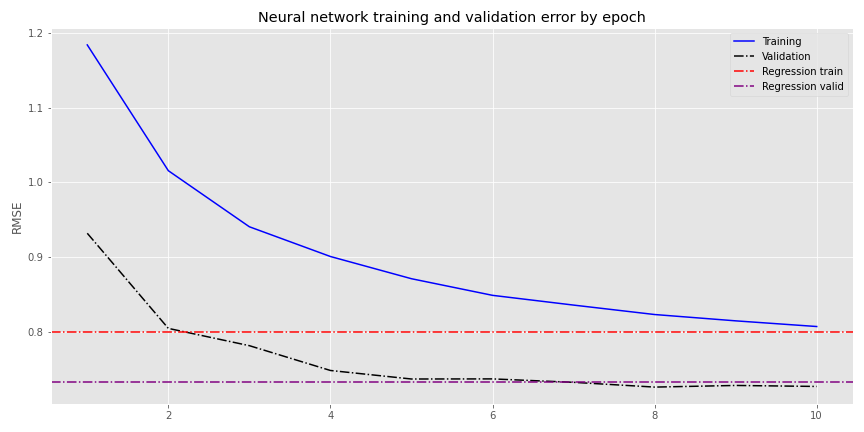Interestingly, the DNN takes longer to reach the same RMSE as the regression than the simple NN. This is likely due to the order of magnitude increase in calculations. However, the DNN converges much faster on the validation data. We’re not exactly sure why this might be the case, but suspect that it’s because of the validation data rather than the model. Importantly, notice that the linear regression’s error is also lower on the validation vs. the training set, confirming that the issue is not so much with the model, but with the time series.

The point of this example is to show three things. First, NNs can approximate the results of a linear regression, with a sufficient number of iterations. Second, since both algorithms end up with lower errors on the validation data, neither algorithm produces a better model. Third, adding complexity, as we did with the DNN, didn’t necessarily improve results.

Given these observations, one can understand why a number of practitioners don’t see the point of applying neural networks to investing. If they don’t produce better results than a linear regression, why use them in the first place? You’re adding complexity with no additional benefit and perhaps some additional cost either in time to learn the algorithms or the money to hire someone to do it for you.

A toy example shouldn’t cloud an open mind, however. The manifest flexibility of a neural network—the ability to model linear and non-linear and higher dimensional relationships—suggests there’s a lot more to consider. That’s for future posts!

In this post, we’ve introduced neural networks and formulated some of the questions we want to answer over this series. Our plan is to apply the major NN architectures not only to typical technical analysis, but also to fundamental and factor investing. We might even introduce sentiment analysis and natural language processing if we don’t go crazy first. If you have any thoughts or opinions on this post or our plan, email us at content at optionstocksmachines dot com. And now, the code!

 Built using R 4.0.3, and Python 3.8.3

# [R]
suppressPackageStartupMessages({
library(tidyverse)
library(tidyquant)
library(reticulate)
})

# [Python]
import warnings
warnings.filterwarnings('ignore')
import numpy as np
import pandas as pd
import statsmodels.api as sm
import matplotlib
import matplotlib.pyplot as plt
import os
os.environ['QT_QPA_PLATFORM_PLUGIN_PATH'] = 'C:/Users/user_name/Anaconda3/Library/plugins/platforms'

plt.style.use('ggplot')
plt.rcParams['figure.figsize'] = (12,6)

# Directory to save images
# Most of the graphs are now imported as png. We've explained why in some cases that was necessary due to the way reticulate plays with Python. But we've also found that if we don't use pngs, the images don't get imported into the emails that go to subscribers.

DIR = "your/image/directory"

def save_fig_blog(fig_id, tight_layout=True, fig_extension="png", resolution=300):
path = os.path.join(DIR, fig_id + "." + fig_extension)
print("Saving figure", fig_id)
if tight_layout:
plt.tight_layout()
plt.savefig(path, format=fig_extension, dip=resolution)

## Create single layer perceptron
from nnv import NNV

layers = [
{'title':'Input', 'units':3, 'color':'blue'},
{'title': 'Output', 'units':1, 'color':'grey'}
]

plt.show()

## Create multi-layer perceptron
layersList = [
{"title":"Input\n", "units": 5, "color": "blue"},
{"title":"Hidden 1\n(ReLU)", "units": 12},
{"title":"Hidden 2\n(ReLU)", "units": 10},
{"title":"Hidden 3\n(ReLU)", "units": 8},
{"title":"Hidden 4\n(ReLU)", "units": 6, "color": 'lightGray'},
{"title":"Output\n(Softmax)", "units": 3,"color": "blue"},
]

plt.show()

## Pull S&P data and process
start = '1970-01-01'
end = '2020-12-31'

sp = dr.DataReader('^GSPC', 'yahoo', start, end)

sp_mon.columns = ['close', '10ma']
sp_mon = sp_mon.rename(index = {'Date':'date'})

sp_mon['ret']= sp_mon['close'].pct_change()

# Graph S&P and 10-month moving average
ax = sp_mon[['close', '10ma']].plot(color=['blue', 'black'], style=['-', '-.'])
plt.legend(['Index', '10-month SMA'])
plt.xlabel("")
plt.ylabel("Index")
ax.set_yticklabels(['{:,}'.format(int(x)) for x in ax.get_yticks().tolist()])
plt.title('S&P 500 with moving average')
save_fig_blog('sp_tf1')
plt.show()

sp_mon['1_mon'] = sp_mon['close'].shift(-1)

## Create train, valid, test split and normalize
norms = sp_mon.apply(lambda x: (x-x.rolling(10).mean())/x.rolling(10).std()).dropna()

X_train = norms.loc[:'1991', ['close', '10ma']]
y_train = norms.loc[:'1991', '1_mon']

X_valid = norms.loc['1991':'2000', ['close', '10ma']]
y_valid = norms.loc['1991':'2000', '1_mon']

X_test = norms.loc['2001':, ['close', '10ma']]
y_test = norms.loc['2001':, '1_mon']

## Show NN architecture
layer_list1 = [
{"title":"Input\n", "units": 2, "color": "blue"},
{"title":"Hidden 1\n(ReLU)", "units": 10},
{"title":"Output\n(None)", "units": 1,"color": "blue"},
]
# save_to_file=DIR+"/mlp_tf1.png"

plt.show()

## Build neural network and train
import tensorflow as tf
from tensorflow import keras

keras.backend.clear_session()
np.random.seed(42)
tf.random.set_seed(42)

model = keras.models.Sequential([
keras.layers.Dense(10, activation='relu', input_shape=X_train.shape[1:]),
keras.layers.Dense(1)
])

model.compile(loss='mean_squared_error', optimizer='sgd')
history = model.fit(X_train, y_train, epochs=20)

# Graph loss function
hist = pd.DataFrame(history.history).apply(lambda x: np.sqrt(x))
hist.index = np.arange(1, len(hist)+1)
hist['loss'].plot(color='blue')
plt.legend('')
plt.xlabel('Epoch')
plt.ylabel('RMSE')
plt.xticks(np.arange(0, 21, 2))
plt.title("Neural network training error by epoch")
save_fig_blog('nn_train_1_tf1')
plt.show()

## Include validation set
keras.backend.clear_session()
np.random.seed(42)
tf.random.set_seed(42)
model = keras.models.Sequential([
keras.layers.Dense(10, activation='relu', input_shape=X_train.shape[1:]),
keras.layers.Dense(1)
])

model.compile(loss='mean_squared_error', optimizer='sgd')
history = model.fit(X_train, y_train, epochs=20, validation_data = (X_valid, y_valid))

# Graph
hist = pd.DataFrame(history.history).apply(lambda x: np.sqrt(x))
hist.index = np.arange(1, len(hist)+1)
ax = hist.plot(color=['blue', 'black'], style=['-', '-.'])
plt.legend(['Training', 'Validation'])
plt.xlabel('Epoch')
plt.ylabel('RMSE')
plt.xticks(np.arange(0, 21, 2))
plt.title("Neural network training and validation error by epoch")
save_fig_blog('nn_train_2_tf1')
plt.show()

## Add early stopping with 100 epochs
keras.backend.clear_session()
np.random.seed(42)
tf.random.set_seed(42)

model = keras.models.Sequential([
keras.layers.Dense(10, activation='relu', input_shape=X_train.shape[1:]),
keras.layers.Dense(1)
])

model.compile(loss="mean_squared_error", optimizer='sgd', metrics=['mape'])
check_pt = keras.callbacks.ModelCheckpoint("tf1_model.h5", save_best_only=True)
early_stop = keras.callbacks.EarlyStopping(patience=2, restore_best_weights=True)
history = model.fit(X_train, y_train, epochs=100,
validation_data=(X_valid, y_valid),
callbacks=[check_pt, early_stop])
model = keras.models.load_model("tf1_model.h5") # rollback to best model

## Comparing NN to linear regression
import statsmodels.api as sm

y = y_train

lin_reg = sm.OLS(y, X).fit()

keras.backend.clear_session()
np.random.seed(42)
tf.random.set_seed(42)

lin_nn = keras.models.Sequential([
keras.layers.Dense(1, input_shape=X_train.shape[1:])
])

lin_nn.compile(loss='mse', optimizer='sgd')

lin_hist = lin_nn.fit(X_train, y_train, epochs=8)

# Graph loss function
lin_hist_df = pd.DataFrame(lin_hist.history).apply(lambda x: np.sqrt(x))
lin_hist_df.index = np.arange(1, len(lin_hist_df)+1)
lin_hist_df.plot(color='blue')
plt.axhline(np.sqrt(lin_reg.mse_resid), color='red', ls = ':')
plt.xlabel('Epoch')
plt.ylabel('RMSE')
plt.title("Neural network training error by epoch")
plt.legend(['Training', 'Linear regression'])
save_fig_blog('nn_vs_lin_reg_tf1')
plt.show()

# Print table of weights, biases, and coefficients
nn_params = np.concatenate((lin_nn.layers.get_weights().astype(np.float),lin_nn.layers.get_weights().flatten().astype(np.float)),axis=0)
lin_reg_params = lin_reg.params.values
pd.DataFrame(np.array([lin_reg_params, nn_params]), columns = ['Intercept', 'Close', 'SMA'],
index = ['Linear', 'Neural Network']).apply(lambda x: round(x,3))
## Build dense NN
keras.backend.clear_session()
np.random.seed(42)
tf.random.set_seed(42)

model = keras.models.Sequential([
keras.layers.Dense(300, activation='relu', input_shape=X_train.shape[1:]),
keras.layers.Dense(100, activation='relu'),
keras.layers.Dense(1)
])

model.compile(loss="mean_squared_error", optimizer='sgd')

check_pt = keras.callbacks.ModelCheckpoint("tf1_dense_model.h5", save_best_only=True)
early_stop = keras.callbacks.EarlyStopping(patience=2, restore_best_weights=True)

history = model.fit(X_train, y_train, epochs=100,
validation_data=(X_valid, y_valid),
callbacks=[check_pt, early_stop])

# Graph loss functions
rmse_train = np.sqrt(lin_reg.mse_resid)
rmse_valid = np.sqrt(np.mean((pred-y_valid)**2))

hist2 = pd.DataFrame(history.history).apply(lambda x: np.sqrt(x))
hist2.index = np.arange(1, len(hist2)+1)

hist2.plot(color = colors, style = styles)

plt.title("Neural network training and validation error by epoch")
plt.ylabel('RMSE')
plt.axhline(rmse_train, color='red', ls = '-.')
plt.axhline(rmse_valid, color='purple', ls = '-.')

plt.legend(labs+['Regression train', 'Regression valid'])
save_fig_blog('dnn_vs_lin_reg_tf1')

plt.show()

1. The search term “forecasting stock prices with neural networks” produced 44,000 results. The search term “forecasting stock prices” produced 752,000 results.↩︎

2. By this we mean, actually implementing the strategy you’re planning to implement. “Trading the plan”↩︎

3. We create this image using the nnv package in Python. Source: R. Cordeiro, “NNV: Neural Network Visualizer”, 2019. [Online]. Available: here [Accessed: 22-February- 2021].↩︎

4. The three main functions are: Sigmoid: $$1/(1 + e^{-x})$$, Hyperbolic Tangent: $$2\sigma(2x) - 1$$, and Rectilinear unit: $$max(0,x)$$. But there are lots of others too like ELU, SELU, leaky this, and squeaky that.↩︎

5. For example, the exclusive or, aka XOR, problem, or, not A and B or A and not B.↩︎

6. As usual, our notation may not be perfectly formal, but we hope we get the point across.↩︎

7. That is, at each neuron we take the partial derivative of the loss function with respect to the weight or bias and then tweak the weight by some small number known as the learning rate.↩︎

8. The initialization step is it’s own area of fertile research with abundance of eponymous initializers.↩︎

9. As Charles Babbage once said, “On two occasions I have been asked [by members of Parliament], ‘Pray, Mr. Babbage, if you put into the machine wrong figures, will the right answers come out?’ I am not able rightly to apprehend the kind of confusion of ideas that could provoke such a question.”↩︎